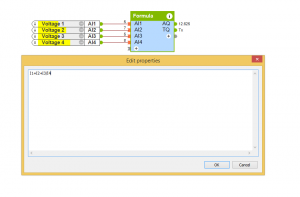# Formula

## INTRODUCTION

The 4 inputs AI1-AI4 are analogue inputs where variables or constants can be used in the calculations.

You can enter the formula for the calculation in the properties of the block. The four inputs are assigned as I1, I2, I3 and I4. The result of the calculation is output at AQ.

If an illegal mathematic operation is performed (for example division by 0) then an error message is output at TQ.

## EXAMPLE

The formula block is useful for calculating the mean of values for example voltages. To do this with the block you would add the voltages and then divide by the total number of voltages.

For example: (I1+I2+I3)/4## SUPPORTED FUNCTIONS

 Function Description + Addition – Subtraction * Multiplication / Division ^ Raise to the power PI Value of Pi (~3.14) ABS Absolute value SQRT Square root SINH Hyperbolic sine COSH Hyperbolic cosine TANH Hyperbolic tangent LN Natural logarithm of a value (base e) LOG Common logarithm (base 10) EXP Exponential function SIN Sine COS Cosine TAN Tangent ARCSIN Arcsine ARCCOS Arccosine ARCTAN Arctangent RAD Radians (2PI) DEG Degrees (360°)Applications:
Level calculations, mean values, heating system calculations, etc.